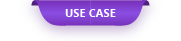We will introduce an example of analyzing the electrical conductivity of carbon nanotubes, which are expected as materials for next-generation semiconductor devices

# Use Case: Calculation of electrical conduction of carbon nanotubes

We will introduce an example of analyzing the electrical conductivity of carbon nanotubes, which are expected as materials for next-generation semiconductor devices, by first-principles calculation. Here, we focused on the effects of carbon defects and cross-linked metals on the electrical conductivity of carbon nanotubes.

#### 1. Computational model

Figure 1 shows the calculation model used. In the electrical conduction calculation, the electrical conduction characteristics are calculated based on the electronic states obtained from the model of the electrode part and the scattering part. In the scattering part, we modeled a carbon nanotube with a chirality of (4,4) and a tube length of 1.73 nm, and based on that model, we created a model with one carbon defect and a model in which Co atoms were cross-linked.

#### 2. Calculation conditions

The electrical conduction calculation was performed using the electrical conduction calculation function pwcond.x of Quantum ESPRESSO (v6.3). The details of the calculation conditions are shown in Table 1.

Table 1 Calculation conditions

itemdetail
PseudopotentialC_ pbe-n-rrkjus_psl. 1.1.0UPF
Co_ pbe-spn-rrkjus_psl. 0.3.1UPF
Cutoff wave function30 Ry
Cutoff electron density225 Ry
k pointScattering part: 1 x 1 x 16
Electrode part: 1 x 1 x 112
Convergence threshold10 -10

#### 3. Calculation result

Figure 3 shows the transmission function representing the electrical conductivity of the CNT model and the results of partial density of states analysis near the Fermi level. The CNT model used this time has a short tube length and the bandgap has not been confirmed, but from Fig. 3 (a), the characteristics of the transmission function that increases stepwise around the vicinity of the Fermi level peculiar to CNT can be reproduced. .. In addition, it was confirmed from Fig. 3 (b) that the distribution of the transmission function corresponds to the distribution of the p-orbital of the C atom showing the π bond of CNT.

Next, Fig. 4 shows the calculation results of the CNT model with carbon defects. Looking at Fig. 4 (a), it was confirmed that the transmission function was smaller overall and the electrical conductivity was lower than in the case without the defect in Fig. 3. Looking at the results of the density of states analysis in Fig. 4 (b), it can be confirmed that the effect of carbon defects appears near the Fermi level compared to Fig. 3 (b), which has no defects. It is thought that the electrical conductivity will decrease.

Next, Fig. 4 shows the calculation results of the electrical conductivity of CNTs with Co-crosslinks. Since it has spin polarization due to the cross-linking of Co atoms, the transmission function and partial density of states were analyzed for both UP spin and DOWN spin. From Fig. 4 (a), it was found that the transmission function of the DOWN spin has a smaller transmission function below the Fermi level than the UP spin, and the electrical conductivity decreases under a finite bias. Looking at the partial density of states in Figures 4 (b) and (c), the d-orbital component of Co is distributed near the Fermi level of the DOWN spin, and the p-orbital component of C interacts with the d-orbital of Co. You can see that the effect of is appearing.